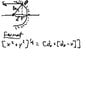# Find equation for shape of reflecting surface

CaptainQuaser
Here is the question, I just need verification, don't know how to make the fancy formulas here so bear with me...

Consider a reflecting surface that produces parallel rays from a point source of light. The axis of the mirror lies on the x-axis which passes through the mirror at x=y=0. The source is at x = d, y=0 and rays of light are produced paralled to the x axis. Using Fermat's Principle, derive the equation of the relecting surface.

I am doing this on my palm pilot right now, so I will try to draw a picture of my thoughts.

#### Attachments

•fermat.JPG
14.9 KB · Views: 370